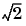Wavelet Toolboxdbaux

Daubechies wavelet filter computation

Syntax

• ```W = dbaux(N,SUMW)
W = dbaux(N)
```

Description

`W = dbaux(N,SUMW)` is the order `N` Daubechies scaling filter such that `sum(W) = SUMW`. Possible values for `N` are 1, 2, 3, ...

 Note    Instability may occur when `N` is too large.

`W = dbaux(N)` is equivalent to `W = dbaux(N,1)`

`W = dbaux(N,0)` is equivalent to `W = dbaux(N,1)`

Examples

• ```% P the "Lagrange à trous" filter for N=2 is explicit
% and given by:
P = [ -1/16 0 9/16 1 9/16 0 -1/16]

P =
-0.0625        0  0.5625  1.0000  0.5625        0 -0.0625

% The db2 Daubechies scaling filter w, is a
% solution of the equation: P = conv(wrev(w),w) * 2.
%
% This filter P is symmetric, easy to generate, and w is
% a minimum phase solution of the previous equation,
% based on the roots of P.
rP = roots(P);

% Retaining only the root inside the unit circle (here it
% is the sixth value of rP), and two roots located at -1,
% we obtain the Daubechies wavelet of order 2:
ww = poly([rP(6) -1 -1]);    % filter construction
ww = ww / sum(ww)            % normalize sum

ww =
0.3415  0.5915  0.1585 -0.0915

% Check that ww is correct and equal to
% the db2 Daubechies scaling filter w.
w = dbaux(2)

w =
0.3415  0.5915  0.1585 -0.0915
```

Algorithm

The algorithm used is based on a result obtained by Shensa (see "References"), showing a correspondence between the "Lagrange à trous" filters and the convolutional squares of the Daubechies wavelet filters.

The computation of the order N Daubechies scaling filter w proceeds in two steps: compute a "Lagrange à trous" filter P, and extract a square root. More precisely:

• P the associated "Lagrange à trous" filter is a symmetric filter of length
4N-1. P is defined by

P = [a(N) 0 a(N-1) 0 ... 0 a(1) 1 a(1) 0 a(2) 0 ... 0 a(N)]

where

•• Then, if w denotes dbN Daubechies scaling filter of sum, w is a square root of P:
•                    P = `conv`(`wrev`(w),w) where w is a filter of length 2N.

The corresponding polynomial has N zeros located at -1 and N-1 zeros less than 1 in modulus.

Note that other methods can be used; see various solutions of the spectral factorization problem in Strang-Nguyen (p. 157).

Limitations

The computation of the `dbN` Daubechies scaling filter requires the extraction of the roots of a polynomial of order `4N`. Instability may occur when `N` is too large.

```dbwavf, wfilters ```cwt dbwavf